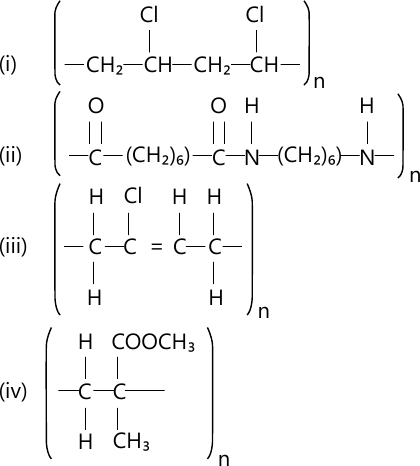MORE IN Engineering Chemistry
RGPV First Year Engineering (Set A) (Semester 1)
Engineering Chemistry
May 2012
Total marks: --
Total time: --
INSTRUCTIONS
(1) Assume appropriate data and state your reasons
(2) Marks are given to the right of every question
(3) Draw neat diagrams wherever necessary

Solve any one question from Q1 & Q2
1 (a) Discuss various methods used for disinfection of water.
7 M
1 (b) Explain with equations and calculate the quantity of lime and soda required to soften 20000 litres of water containing:
(i) 219 ppm of magnesium bicarbonate and 234 ppm of sodium chloride.
(ii) 36 ppm of Mg2+ ions and 18.3 ppm of HCO3-
(iii) 1⋅5 ppm of free acid 144 ppm of sulphate ions and 71 ppm of chloride ions.
7 M

2 (a) Draw a well labelled diagram of Ion-Exchange resin method for softening hard water. How demineralised water is different from distilled water?
7 M
2 (b) What is scale? How is it formed? Write disadvantages and methods of removal of scale in boilers.
7 M

Solve any one question from Q3 & Q4
3 (a) Mention the criteria for selecting good fuel. What are the advantages of liquid fuels over solid fuels?
7 M
3 (b) Explain proximate analysis of coal giving the significance.
7 M

4 (a) What is knocking? How is antiknocking characteristics of a fuel improved?
7 M
4 (b) 100 kg of a liquid hydrocarbon fuel containing 90% C by wt. Is brunt with:
(i) theoretical amount of air.
(ii) 20% excess of the theoretically required amount of air.
Calculate the volumetric composition of the products in each case.
7 M

Solve any one question from Q5 & Q6
5 (a) What are refractories? How significant are the properties of RUL, thermal conductivity and thermal spalling, when the refractories are put to industrial use?
7 M
5 (b) Define the terms lubrication and lubricant. Write the functions of lubricant. Discuss the basic principle of lubrication.
7 M

6 (a) Describe the manufacture of Portland Cement with the chemical reactions involved in it. Discuss the role the gypsum in Portland Cement.
7 M
6 (b) Define the following terms and explain their significance in case of lubricant oil:
(i) Flash point and fire point
(ii) Saponification number
(ii) S.E.N.
7 M

Solve any one question from Q7 & Q8
7 (a) Define the term polymer. Give classification of polymers. Discuss the role of functionality in polymerisation.
7 M
7 (b) What is rubber? Write its different types. What is the basic chemical unit present in natural rubber and what happens when the configuration of it changes to geometrical isomer?
7 M

8 (a) Give the separation and uses of the following:
(i) SBR
(ii) Bakelite
7 M
8 (b) Write the names of repeating units in the following:4 M
8 (c) Distinguish between thermoplastic and termosetting resins.
3 M

Solve any one question from Q9 & Q10
9 (a) Name and state the basic laws of photochemistry. How the laws may be used to determine the concentration in solution?
7 M
9 (b) (i) 100 ml of water sample on titration with N/50 H2SO4 using phenolphthalein as indicator gave the end point when 10 ml of acid were run. 100 ml of same water sample separately required 10 ml of acid to obtain Methly orange end point. Find the type and extent of alkalinity.
4 M
9 (b) (ii) 100 ml of water sample was treated with excess of 10% KI solution and then titrated against N/10 hypo solution using starch as indicator. 2⋅5 ml of hypo was used for starch end point. Calculate the amount of free chlorine in water.
3 M

10 (a) Discuss principle and working of gas chronatography. Give its important applications.
7 M
10 (b) (i) 100 ml of standard hard water containing 1⋅5 mg of pure CaCO3 per ml consumed 44 ml of EDTA. 50 ml of a water sample consumed 20 ml of the same EDTA solution, using eriochrome Black-T as indicator. Calculate total hardness of water in ppm and °CI units.
4 M
10 (b) (ii) 200 ml of a water sample contains 560 ppm of dissolved oxygen. After 5 days the dissolved oxygen value becomes 335 ppm after the sample has been diluted to 600 ml. Calculate the BOD of the sample.
3 M

More question papers from Engineering Chemistry